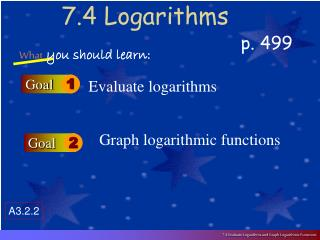DownloadDownload Presentation7.4 Logarithms

# 7.4 Logarithms

Download Presentation## 7.4 Logarithms

- - - - - - - - - - - - - - - - - - - - - - - - - - - E N D - - - - - - - - - - - - - - - - - - - - - - - - - - -
##### Presentation Transcript

1. 7.4 Logarithms Whatyou should learn: Goal 1 Evaluate logarithms p. 499 Graph logarithmic functions Goal 2 A3.2.2 7.4 Evaluate Logarithms and Graph Logarithmic Functions

2. Evaluating Log Expressions • We know 22 = 4 and 23 = 8 • But for what value of y does 2y = 6? • Because 22 < 6 < 23 you would expect the answer to be between 2 & 3. • To answer this question exactly, mathematicians defined logarithms.

3. Definition of Logarithm to base a • Let a & x be positive numbers & a ≠ 1. • The logarithm of x with base a is denoted by logax and is defined: • logax = y iff ay = x • This expression is read “log base a of x” • The function f(x) = logax is the logarithmic function with base a.

4. The definition tells you that the equations logax = y and ay = x are equivilant. • Rewriting forms: • To evaluate log3 9 = x ask yourself… • “Self… 3 to what power is 9?” • 32 = 9 so…… log39 = 2

5. log216 = 4 log1010 = 1 log31 = 0 log10 .1 = -1 log2 6 ≈ 2.585 24 = 16 101 = 10 30 = 1 10-1 = .1 22.585 = 6 Log formExp. form

6. log381 = Log5125 = Log4256 = Log2(1/32) = 3x = 81 5x = 125 4x = 256 2x = (1/32) Evaluate without a calculator 4 3 4 -5

7. Evaluating logarithms now you try some! 2 • Log 4 16 = • Log 5 1 = • Log 4 2 = • Log 3 (-1) = • (Think of the graph of y=3x) 0 ½ (because 41/2 = 2) undefined

8. You should learn the following general forms!!! • Log a 1 = 0 because a0 = 1 • Log a a = 1 because a1 = a • Log a ax = x because ax = ax

9. Natural logarithms • log e x = ln x • ln means log base e

10. Common logarithms • log 10 x = log x • Understood base 10 if nothing is there.

11. Common logs and natural logs with a calculator log10 button ln button

12. g(x) = log b x is the inverse of • f(x) = bx • f(g(x)) = x and g(f(x)) = x • Exponential and log functions are inverses and “undo” each other

13. So: g(f(x)) = logbbx = x • f(g(x)) = blogbx = x • 10log2 = • Log39x = • 10logx = • Log5125x = 2 Log3(32)x = Log332x= 2x x 3x

14. Finding Inverses • Find the inverse of: • y = log3x • By definition of logarithm, the inverse is y=3x • OR write it in exponential form and switch the x & y! 3y = x 3x = y

15. Finding Inverses cont. • Find the inverse of : • Y = ln (x +1) • X = ln (y + 1) Switch the x & y • ex = y + 1 Write in exp form • ex – 1 = y solve for y

16. Assignment

17. Graphs of logs • y = logb(x-h)+k • Has vertical asymptote x=h • The domain is x>h, the range is all reals • If b>1, the graph moves up to the right • If 0<b<1, the graph moves down to the right

18. Graph y =log5(x+2) • Plot easy points (-1,0) & (3,1) • Label the asymptote x=-2 • Connect the dots using the asymptote. X=-2

19. Assignment